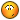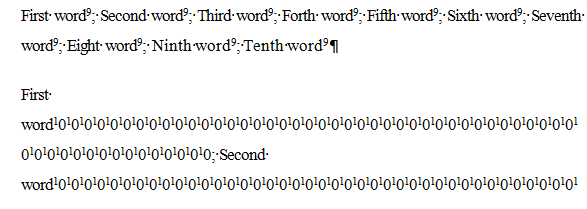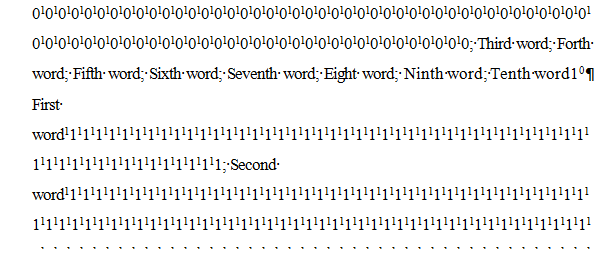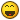# Add number based on InputBox() in Word Document

## Recommended Posts

Hi @Subz,

I know this concern was already solved previously, but as far as what I've gathered information from my Dept. they need to have it their choice when adding numbers in section headings and then the rest of numbers will follow "+1".

I tried changing the code you've shared but I failed (Not really good yet with Word). Sorry if I re-opened it again.

Here's what I need:

`\$iInput = InputBox("Numeric Count Only", "Number to Start:","","",1,0)`

Others are welcome for suggestions. Much appreciated!KS15

Programming is "To make it so simple that there are obviously no deficiencies" or "To make it so complicated that there are no obvious deficiencies" by C.A.R. Hoare.

##### Share on other sites

Did you mean something like this:

```#include <Array.au3>
#include <Word.au3>
Local \$oWord = _Word_Create()
Local \$sDocument = @ScriptDir & "\WordFile.docx"
Local \$oDoc = _Word_DocOpen(\$oWord, \$sDocument, Default, Default, True)
Local \$oFindRange, \$oLastRange, \$oNextRange, \$i = 0
Local \$x = InputBox("Numeric Count Only", "Number to Start:","","",1,0)
\$oWord.Selection.HomeKey(\$wdStory)
For \$j = 1 To \$oDoc.Paragraphs.Count
If StringInStr(\$oDoc.Paragraphs(\$j).Range.text, ", ") And \$oDoc.Paragraphs(\$j).Range.Bold = True Then
\$i += 1
If \$i = 1 Then
\$oNextRange = \$oDoc.Paragraphs(\$j + 1).Range
\$oLastRange = \$oDoc.Paragraphs(\$i).Range
ElseIf \$i > 1 Then
\$oNextRange = \$oDoc.Paragraphs(\$j + 1).Range
\$oDoc.Paragraphs(\$j).Range.Cut
\$oRange = \$oDoc.Range(\$oLastRange.End, \$oLastRange.End)
\$oRange.Paste
\$oLastRange = \$oDoc.Paragraphs(\$i).Range
EndIf
\$oDoc.Paragraphs(\$i).Range.InsertBefore(\$x)
\$oDoc.Range(\$oDoc.Paragraphs(\$i).Range.Start, \$oDoc.Paragraphs(\$i).Range.Start + 1).Font.Superscript = True

\$oFindRange = _Word_DocFind(\$oDoc, ", ", -1, \$oDoc.Paragraphs(\$j).Range)
If Not @error Then
\$oRange = _Word_DocRangeSet(\$oDoc, \$oFindRange, Default, Default, \$wdCharacter, 0)
\$oRange.Delete
\$oRange.InsertBreak(\$wdLineBreak)
EndIf
EndIf
If IsObj(\$oNextRange) And StringInStr(\$oNextRange.text, "; ") Then
\$oFindRange = \$oNextRange
\$oFindRange.Find.ClearFormatting
\$oFindRange.Find.text = "; "
\$iEndRange = \$oFindRange.End
Do
\$oFindRange.Find.Execute
If \$oFindRange.Find.Found Then
\$oFindRange.Text = \$x & \$oFindRange.Text
\$oFindRange.Start = \$oFindRange.Start
\$oFindRange.End = \$oFindRange.Start + 1
\$oFindRange.Font.Superscript = True
\$oFindRange.Start += 2
\$oFindRange.End = \$iEndRange
EndIf
Until \$oFindRange.Find.Found = False
\$oDoc.Range(\$oDoc.Paragraphs(\$i + \$i).Range.Start, \$oDoc.Paragraphs(\$i + \$i).Range.End - 1).InsertAfter(\$x)
\$oDoc.Range(\$oDoc.Paragraphs(\$i + \$i).Range.End - 2, \$oDoc.Paragraphs(\$i + \$i).Range.End - 1).Font.Superscript = True
\$x += 1
EndIf
Next```

##### Share on other sites

Sorry for late reply, I was off for weekend here in our country. Anyway, I have tested your code and the attached is the output.

After number 9, when inserting number 10 all characters within the paragraph were changed like "1010101010" and so on...Programming is "To make it so simple that there are obviously no deficiencies" or "To make it so complicated that there are no obvious deficiencies" by C.A.R. Hoare.

##### Share on other sites

Can you try:

```#include <Array.au3>
#include <Word.au3>
Local \$oWord = _Word_Create()
Local \$sDocument = @ScriptDir & "\WordFile.docx"
Local \$oDoc = _Word_DocOpen(\$oWord, \$sDocument, Default, Default, True)
Local \$oFindRange, \$oLastRange, \$oNextRange, \$i = 0
Local \$x = InputBox("Numeric Count Only", "Number to Start:","","",1,0)
\$oWord.Selection.HomeKey(\$wdStory)
For \$j = 1 To \$oDoc.Paragraphs.Count
If StringInStr(\$oDoc.Paragraphs(\$j).Range.text, ", ") And \$oDoc.Paragraphs(\$j).Range.Bold = True Then
\$i += 1
If \$i = 1 Then
\$oNextRange = \$oDoc.Paragraphs(\$j + 1).Range
\$oLastRange = \$oDoc.Paragraphs(\$i).Range
ElseIf \$i > 1 Then
\$oNextRange = \$oDoc.Paragraphs(\$j + 1).Range
\$oDoc.Paragraphs(\$j).Range.Cut
\$oRange = \$oDoc.Range(\$oLastRange.End, \$oLastRange.End)
\$oRange.Paste
\$oLastRange = \$oDoc.Paragraphs(\$i).Range
EndIf
\$oDoc.Paragraphs(\$i).Range.InsertBefore(\$x)
\$oDoc.Range(\$oDoc.Paragraphs(\$i).Range.Start, \$oDoc.Paragraphs(\$i).Range.Start + StringLen(\$x)).Font.Superscript = True

\$oFindRange = _Word_DocFind(\$oDoc, ", ", -1, \$oDoc.Paragraphs(\$j).Range)
If Not @error Then
\$oRange = _Word_DocRangeSet(\$oDoc, \$oFindRange, Default, Default, \$wdCharacter, 0)
\$oRange.Delete
\$oRange.InsertBreak(\$wdLineBreak)
EndIf
EndIf
If IsObj(\$oNextRange) And StringInStr(\$oNextRange.text, "; ") Then
\$oFindRange = \$oNextRange
\$oFindRange.Find.ClearFormatting
\$oFindRange.Find.text = "; "
\$iEndRange = \$oFindRange.End
Do
\$oFindRange.Find.Execute
If \$oFindRange.Find.Found Then
\$oFindRange.Text = \$x & \$oFindRange.Text
\$oFindRange.Start = \$oFindRange.Start
\$oFindRange.End = \$oFindRange.Start + StringLen(\$x)
\$oFindRange.Font.Superscript = True
\$oFindRange.Start += StringLen(\$x) + 2
\$oFindRange.End = \$iEndRange + StringLen(\$x)
EndIf
Until \$oFindRange.Find.Found = False
\$oDoc.Range(\$oDoc.Paragraphs(\$i + \$i).Range.Start, \$oDoc.Paragraphs(\$i + \$i).Range.End - 1).InsertAfter(\$x)
\$oDoc.Range(\$oDoc.Paragraphs(\$i + \$i).Range.End - (StringLen(\$x) + 1), \$oDoc.Paragraphs(\$i + \$i).Range.End - 1).Font.Superscript = True
\$x += 1
EndIf
Next```

##### Share on other sites

@Subz, It did do want i wanted. That worked, thank you so much subz. You the best....Programming is "To make it so simple that there are obviously no deficiencies" or "To make it so complicated that there are no obvious deficiencies" by C.A.R. Hoare.

##### Share on other sites

@Subz, Sorry for this, but when i tried having around 50 entries in word document and it causes the below error during the run time.

"D:\Program\Autoit\Subz.au3" (53) : ==> The requested action with this object has failed.:
\$oDoc.Range(\$oDoc.Paragraphs(\$i + \$i).Range.Start, \$oDoc.Paragraphs(\$i + \$i).Range.End - 1).InsertAfter(\$x)
\$oDoc.Range(\$oDoc^ ERROR

I'm not sure what causes the error or it's the limitation of the code? tried fixing it but can't manage so far.Thanks.

Programming is "To make it so simple that there are obviously no deficiencies" or "To make it so complicated that there are no obvious deficiencies" by C.A.R. Hoare.

##### Share on other sites

Was it at the last paragraph that it failed or somewhere in between?

##### Share on other sites

It's somewhere in between, it will just stop and flagged this as error.

Programming is "To make it so simple that there are obviously no deficiencies" or "To make it so complicated that there are no obvious deficiencies" by C.A.R. Hoare.

##### Share on other sites

Can you post the doc and also which number you want to start at?  I just did 500 and couldn't get an error starting from 1, I then did 100 lines starting from 50 again without error so not really sure why you're having issues.

##### Share on other sites

That's weird, i tried having it around 200 and it crashes the code. But when having it around 25 the code is fine.

I have here attached the real scenario of the document of what we've been doing. Have a try.

Edited by KickStarter15
Edit: I started from 1 to the end of the paragraph.

Programming is "To make it so simple that there are obviously no deficiencies" or "To make it so complicated that there are no obvious deficiencies" by C.A.R. Hoare.

##### Share on other sites

Note: Noticed that some only had 1 name (i.e. no semi-colons) which was breaking it, hopefully I've fixed that
Some of the names also have commas rather than semi colons so that would need to be fixed.

Anyway this worked for me, with the text document above.

```#include <Array.au3>
#include <Word.au3>
Local \$sDocument = @ScriptDir & "\WordFile.docx"
Local \$oWord = _Word_Create()
Local \$oDoc = _Word_DocOpen(\$oWord, \$sDocument, Default, Default, True)
Local \$oCurrentRange, \$oNextRange, \$iParagraphCount = 0
Local \$iCount = InputBox("Numeric Count Only", "Number to Start:","1","",1,0)
\$iCount -= 1
\$oWord.Selection.HomeKey(\$wdStory)
For \$i = 1 To \$oDoc.Paragraphs.Count
If StringInStr(\$oDoc.Paragraphs(\$i).Range.text, ", ") And \$oDoc.Paragraphs(\$i).Range.Bold = True Then
\$iParagraphCount += 1
\$iCount += 1
If \$iParagraphCount = 1 Then
\$oLastRange = \$oDoc.Paragraphs(\$i).Range
\$oNextRange = \$oDoc.Paragraphs(\$i + 1).Range
ElseIf \$iParagraphCount > 1 Then
\$oCurrentRange = \$oDoc.Paragraphs(\$i).Range
\$oCurrentRange.Cut
\$oLastRange = \$oDoc.Range(\$oLastRange.End, \$oLastRange.End)
\$oLastRange.Paste
\$oNextRange = \$oDoc.Paragraphs(\$i + 1).Range
EndIf
\$oDoc.Range(\$oLastRange.Start, \$oLastRange.End).InsertBefore(\$iCount)
\$oDoc.Range(\$oLastRange.Start, \$oLastRange.Start + StringLen(\$iCount)).Font.Superscript = True

EndIf
If IsObj(\$oNextRange) Then ;And StringInStr(\$oNextRange.text, "; ") Then
If StringInStr(\$oNextRange.text, "; ") Then
\$oNextRange.Find.ClearFormatting
\$oNextRange.Find.text = "; "
Do
\$oNextRange.Find.Execute
If \$oNextRange.Find.Found Then
\$oNextRange.Text = \$iCount & \$oNextRange.Text
\$oDoc.Range(\$oNextRange.Start, \$oNextRange.Start + StringLen(\$iCount)).Font.Superscript = True
\$oNextRange.Start = \$oNextRange.Start + (StringLen(\$iCount) + 2)
\$oNextRange.End = \$oDoc.Paragraphs(\$i + 1).Range.End
EndIf
Until \$oNextRange.Find.Found = False
EndIf
If StringRight(\$oNextRange.Text, StringLen(\$iCount) + 1) <> \$iCount Then
\$oDoc.Range(\$oNextRange.End - (StringLen(\$iCount) + 1), \$oNextRange.End - 1).InsertAfter(\$iCount)
\$oDoc.Range(\$oNextRange.End - (StringLen(\$iCount) + 1), \$oNextRange.End - 1).Font.Superscript = True
EndIf
EndIf
;If \$iParagraphCount = 5 Then ExitLoop
Next
\$oWord.Selection.HomeKey(\$wdStory)
For \$i = 1 To \$oDoc.Paragraphs.Count
If StringInStr(\$oDoc.Paragraphs(\$i).Range.text, ", ") And \$oDoc.Paragraphs(\$i).Range.Bold = True Then
\$oFindRange = \$oDoc.Paragraphs(\$i).Range
\$oFindRange.Find.ClearFormatting
\$oFindRange.Find.Text = ", "
Do
\$oFindRange.Find.Execute
If \$oFindRange.Find.Found Then
\$oFindRange.Delete
\$oFindRange.InsertBreak(\$wdLineBreak)
EndIf
Until \$oFindRange.Find.Found = False
EndIf
Next```

##### Share on other sites

@Subz, Thank you so much for your help. It is now working great.But I'll try this with big file later on. Thank you very much subz.....

KS15

Programming is "To make it so simple that there are obviously no deficiencies" or "To make it so complicated that there are no obvious deficiencies" by C.A.R. Hoare.

## Create an account

Register a new account

• ### Recently Browsing   0 members

×

• Wiki

• Back

• #### Beta

• Git
• FAQ
• Our Picks
×
• Create New...